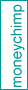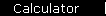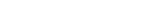Price to Earnings Ratio

Although discounted cash flows is the correct way to value a company, people naturally like to use simpler rules of thumb. The P/E ratio is the most popular because it's easy to understand. If you buy stock at a P/E ratio of 15, say, then it will take 15 years for the company's earnings to add up to your original purchase price - 15 years to "pay you back". That's assuming that the company is already in its "mature" stage, where earnings are constant.

Let's make that last paragraph a little more accurate. If you actually use the discounted cash flows formula on a zero growth company, you find that its fair P/E ratio equals 1/R, where R is the discount rate. So, using a discount rate of 11%, you find that the fair P/E for a mature company is 9.09.

Of course, you'd be willing to pay a higher P/E ratio if earnings were growing - the payback time would be quicker. And you'd want to pay less if future earnings looked risky to you for some reason.

This calculator lets you find the relationship between growth rate and the fair P/E ratio. (It's the same calculator as before, but rearranged to express the answer as a P/E ratio rather than a share price.)

 Growth Assumptions
Earnings are expected to grow at a rate of % annually
for the next years,
before leveling off to an annual growth rate of % thereafter.
 Discount Rate
Return available on an appropriate market benchmark investment (like the S&P 500):   %

 Results
Fair P/E Ratio:

(One thing you should try is setting the growth rate to zero and the discount rate to 11%, to make sure that the fair P/E ratio really is 9.09.)

home  |  article  |  glossary  |  calculator  |  about us  |  booksValuation IntroA Little TheoryDCF CalculatorP/E RatioP/S RatioPEG RatioGraham FormulaDividend DiscountBuffett Formula (?)CAPM CalculatorBooks & LinksValuation FormulaStock Market PredictionsMeasuring Investment ReturnsStock Market CAGR